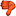CodeGuru Home VC++ / MFC / C++ .NET / C# Visual Basic VB Forums Developer.com

# Thread: I am getting error in python programming question(PLs have a look)

1.BannedJoin Date
Feb 2022
Posts
44

## I am getting error in python programming question(PLs have a look)

Below is question, solution, and error i get when i run solution of this question below.

Q-

Write a program that repeatedly prompts a user for integer numbers until the user enters 'done'. Once 'done' is entered, print out the largest and smallest of the numbers. If the user enters anything other than a valid number catch it with a try/except and put out an appropriate message and ignore the number. Enter 7, 2, bob, 10, and 4 and match the output below.

SOlution-

largest=none
smallest=none
while true:
inp=input("enter number")
if inp=="done": break
try:
num=float(inp)
except:
print("Invalid Input")
continue
if smallest is none:
smallest=num
if num > largest:
largest=num
elif num < smallest:
smallest=num
def done(largest,smallest)
print("maximum is",int(largest))
print("minimun is",int(smallest))
done(largest,smallest)

error-

ERROR: C:\Users\Wali\Desktop\Py4e>ex5.2.py
File "C:\Users\Wali\Desktop\Py4e\ex5.2.py", line 3
while true:
IndentationError: unexpected indent2.MemberJoin Date
Nov 2018
Posts
60

## Re: I am getting error in python programming question(PLs have a look)Originally Posted by existenceproductBelow is question, solution, and error i get when i run solution of this question below.

Q-

Write a program that repeatedly prompts a user for integer numbers until the user enters 'done'. Once 'done' is entered, print out the largest and smallest of the numbers. If the user enters anything other than a valid number catch it with a try/except and put out an appropriate message and ignore the number. Enter 7, 2, bob, 10, and 4 and match the output below.

SOlution-

largest=none
smallest=none
while true:
inp=input("enter number")
if inp=="done": break
try:
num=float(inp)
except:
print("Invalid Input")
continue
if smallest is none:
smallest=num
if num > largest:
largest=num
elif num < smallest:
smallest=num
def done(largest,smallest)
print("maximum is",int(largest))
print("minimun is",int(smallest))
done(largest,smallest)

error-

ERROR: C:\Users\Wali\Desktop\Py4e>ex5.2.py
File "C:\Users\Wali\Desktop\Py4e\ex5.2.py", line 3
while true:
IndentationError: unexpected indent
Repost bot - original is here.
https://www.reddit.com/r/learnpython...n_programming/
Last edited by 2kaud; February 4th, 2023 at 05:21 AM.3. ## Re: I am getting error in python programming question(PLs have a look)

@existenceproduct - why repost a question from 3 years ago that has already been answered??####Posting Permissions

• You may not post new threads
• You may not post replies
• You may not post attachments
• You may not edit your posts
•